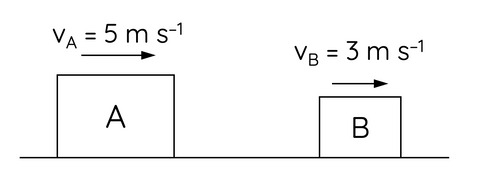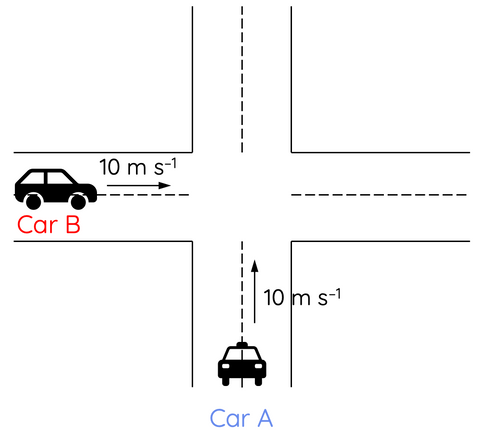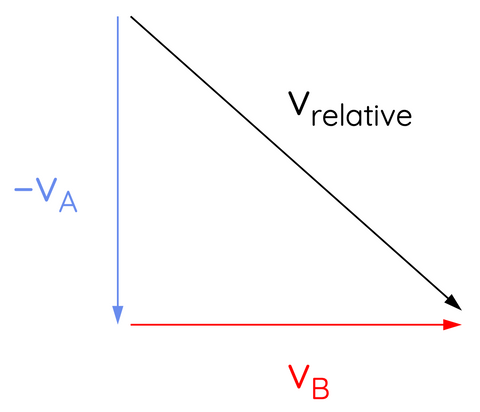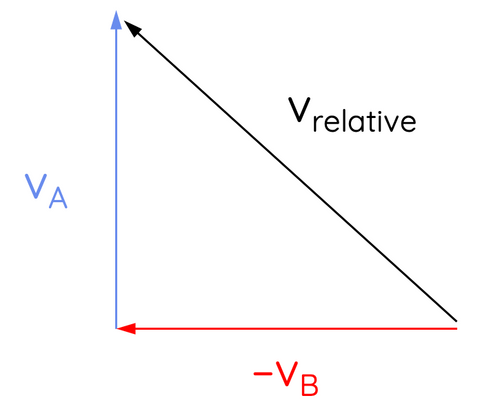# Relative Velocity in One and Two Dimensions

This topic is part of the HSC Physics course under the section Motion on a Plane

### HSC Physics Syllabus

• calculate the relative velocity of two objects moving along the same line using vector analysis
• describe and analyse, using vector analysis, the relative positions and motions of one object relative to another object on a plane (ACSPH061)
• analyse the relative motion of objects in two dimensions in a variety of situations, for example:
– a boat on a flowing river relative to the bank
– two moving cars
– an aeroplane in a crosswind relative to the ground (ACSPH060, ACSPH132)

### Relative Velocity in One Dimension

Relative velocity is the velocity of an object with respect to another object or reference frame. In one-dimensional scenarios, this can be visualised as objects moving along a straight line. The relative velocity is given by the difference between the velocity of the object and the velocity of the reference frame.

$$v_{relative}=v_{object}-v_{reference}$$

Let's consider two objects, A and B, moving along the same straight line, where A has a velocity of v_A and B has a velocity of v_B. The relative velocity of B with respect to A is given by the difference between the velocities of B and A:

$$v_{BA}=v_B-v_A$$For example, if A is moving to the right with a velocity of 5 m/s and B is moving to the right with a velocity of 3 m/s, then the relative velocity of B with respect to A is:

$$v_{BA}=3-5=-2 m s^{-1}$$

The negative sign indicates that B is moving to the left at 2 m/s relative to A. From the perspective of A, B appears to be moving to the left.If two objects are moving in different directions, the relative velocity is still the difference between their velocities.

If A is moving to the right with a velocity of 5 m/s and B is moving to the left with a velocity of 3 m/s, then the relative velocity of B with respect to A is:

$$v_{BA}=-3 - 5= -8 m s^{-1}$$

The positive sign indicates that B is to the left at 8 m/s relative to A. From the perspective A, B appears to be moving to the left at much greater speed than 3 m/s.

### Relative Velocity in Two Dimensions

In two-dimensional scenarios, relative velocity becomes a bit more complex. We need to consider the velocities of the objects in both the x- and y-directions.Let's consider two cars, A and B. Car A is moving north at 10 m/s while car B is moving to the east at 10 m/s.

The velocity of car B relative to car A is given by:

$$v_{BA}=v_B - v_A$$

However, we cannot subtract the magnitudes directly because they are in different directions. Instead, we should draw a diagram to visualise the magnitude and direction of the relative velocity vector.The difference between v_B and v_A is equivalent to the sum of v_B and -v_A. Therefore, the relative velocity vector can be formed by finding the resultant vector between v_B and -v_A.

The magnitude of the relative velocity is calculated using Pythagorean theorem:

$$v_{relative}^2=10^2+10^2$$

$$v_{relative}=\sqrt{10^2+10^2}$$

$$v_{relative}=14 \hspace{1mm} m s^{-1}$$

The direction of the relative velocity is calculated using trigonometry:

$$\tan{\theta}=\frac{10}{10}$$

$$\theta = 45º$$

Therefore the velocity of car B relative to car A is 14 m/s 45 degrees above the x-axis or south east.

What about the velocity of car A relative to car B?

The velocity of car a relative to car B is given by:

$$v_{AB}=v_A - v_B$$

This can be visualised as the sum of vectors v_A and -v_B:The magnitude of the relative velocity is calculated using Pythagorean theorem:

$$v_{relative}^2=10^2+10^2$$

$$v_{relative}=\sqrt{10^2+10^2}$$

$$v_{relative}=14 \hspace{1mm} m s^{-1}$$

The direction of the relative velocity is calculated using trigonometry:

$$\tan{\theta}=\frac{10}{10}$$

$$\theta = 45º$$

Therefore the velocity of car A relative to car B is 14 m/s 45 degrees above the x-axis or north east. In other words, the velocity of car A relative to car B has the same magnitude as the velocity of car B relative to A but a different direction.